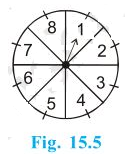# A game of chance consists of spinning an arrow which comes to rest pointing at one of the numbers 1, 2, 3, 4, 5, 6, 7, 8 (see Fig.) , and these are equally likely outcomes. What is the probability that it will point at (i) 8? (ii) an odd number? (iii) a number greater than 2? (iv) a number less than 9?Given that

A game of chance consists of spinning an arrow which comes to rest pointing at one of the numbers 1, 2, 3, 4, 5, 6, 7, 8

Find out

The probability that it will point at

(i) 8?

(ii) an odd number?

(iii) a number greater than 2?

(iv) a number less than 9?

Solution

Total number of possible outcomes = 8

P(E) = (Number of favourable outcomes/ Total number of outcomes)

(i) Total number of favourable events (i.e. 8) = 1

∴ P (pointing at 8) = ⅛ = 0.125

(ii) Total number of odd numbers = 4 (1, 3, 5 and 7)

P (pointing at an odd number) = 4/8 = ½ = 0.5

(iii) Total numbers greater than 2 = 6 (3, 4, 5, 6, 7 and 8)

P (pointing at a number greater than 4) = 6/8 = ¾ = 0.75

(iv) Total numbers less than 9 = 8 (1, 2, 3, 4, 5, 6, 7, and 8)

P (pointing at a number less than 9) = 8/8 = 1

The probability that it will point at

(i) 8 = 0.125

(ii) an odd number = 0.5

(iii) a number greater than 2 = 0.75

(iv) a number less than 9= 1